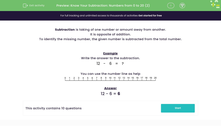# Know Your Subtraction: Subtract Numbers Less Than 20

In this worksheet, students will review their subtraction skills using the number range 0 to 20.Key stage:  KS 1

Curriculum topic:   Number: Addition and Subtraction

Curriculum subtopic:   Add and Subtract to 20

Popular topics:   Subtraction worksheets

Difficulty level:#### Worksheet Overview

Subtraction is the taking of one number or amount away from another.

It is the opposite of addition.

To identify the missing number, the given number is subtracted from the total number.

Example

Write the answer to the subtraction.

 12 - 6 = ?12 - 6 = 6

If you use the number line, pop your finger on the number 12 and move it back to the left 6 places. You should get to 6.

Remember,  you always move to the left on a number line when you are subtracting.Are you ready to have a go at some questions?### What is EdPlace?

We're your National Curriculum aligned online education content provider helping each child succeed in English, maths and science from year 1 to GCSE. With an EdPlace account you’ll be able to track and measure progress, helping each child achieve their best. We build confidence and attainment by personalising each child’s learning at a level that suits them.

Get started